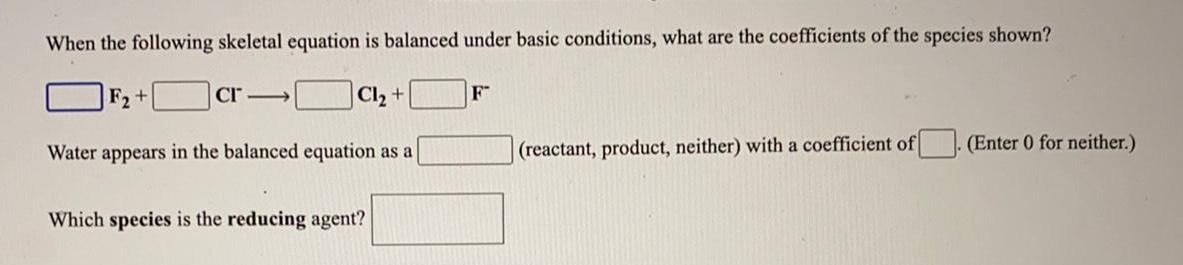Question:

# When the following skeletal equation is balanced under basic

Last updated: 8/5/2022When the following skeletal equation is balanced under basic conditions, what are the coefficients of the species shown? F2 + CI Cl₂ + F- Water appears in the balanced equation as a(reactant, product, neither) with a coefficient of(Enter 0 for neither.) Which species is the reducing agent?